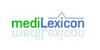### AbohmThe abohm is the basic unit of electrical resistance in the emu-cgs system of units (emu stands for `electromagnetic units`). One abohm is equal to 10-9 ohms in the SI system of units; one abohm is a nanoohm. The emu-cgs (or `electromagnetic cgs`) units are one of several systems of electromagnetic units within the centimetre gram second syste...
Found on http://en.wikipedia.org/wiki/Abohm

### abohmnoun a unit of resistance equal to a billionth of an ohm
Found on https://www.encyclo.co.uk/local/20974

### abohmElectromagnetic unit of resistance equal to 10-9 ohm. ... (05 Mar 2000) ...
Found on http://www.encyclo.co.uk/local/20973

### AbohmThe CGS unit of electrical resistance equal to 1.0x10-9Ohms.ttle='Abohm';xiunt='ohm';yiunt='abohm';mconv=1e-9;cconv=0.0;
Found on http://www.diracdelta.co.uk/science/source/a/b/abohm/source.html

### abohmType: Term Pronunciation: ab′ōm Definitions: 1. Electromagnetic unit of resistance equal to 10−9 ohm.
Found on http://www.medilexicon.com/medicaldictionary.php?t=136

### abohm[n] - a unit of resistance equal to a billionth of an ohm
Found on http://www.webdictionary.co.uk/definition.php?query=abohm
No exact match found